GeeksforGeeks App
Open AppBrowser
Continue

## Related Articles

• NCERT Solutions for Class 9 Maths

# Class 9 NCERT Solutions- Chapter 9 Areas of Parallelograms And Triangles – Exercise 9.3 | Set 2

### Question 11. In Figure, ABCDE is a pentagon. A line through B parallel to AC meets DC produced at F.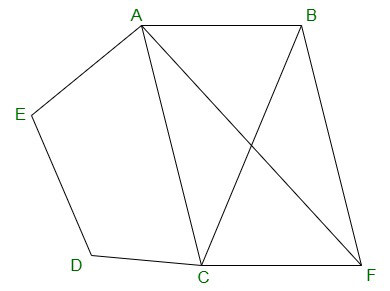### Show that

(i) ar(ΔACB) = ar(ΔACF)

(ii) ar(AEDF) = ar(ABCDE)

Solution:

(i) ΔACB and ΔACF lie on the same base AC and between the same parallels AC and BF.

Therefore,

ar(ΔACB) = ar(ΔACF)

(ii) ar(ΔACB) = ar(ΔACF)

=> ar(ΔACB)+ar(ΔACDE) = ar(ΔACF)+ar(ΔACDE)

=> ar(ABCDE) = ar(AEDF)

### Question 12. A villager Itwaari has a plot of land of the shape of a quadrilateral. The Gram Panchayat of the village decided to take over some portion of his plot from one of the corners to construct a Health Centre. Itwaari agrees to the above proposal with the condition that he should be given equal amount of land in lieu of his land adjoining his plot so as to form a triangular plot. Explain how this proposal will be implemented.

Solution: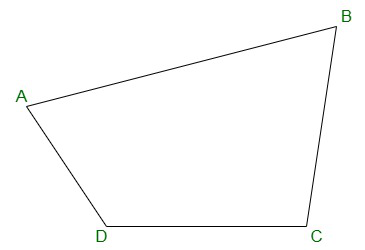Let us assume ABCD be the plot of the land of the shape of a quadrilateral.

Construction:

Join the diagonal BD.

Draw AE parallel to BD.

Join BE, that intersected AD at O.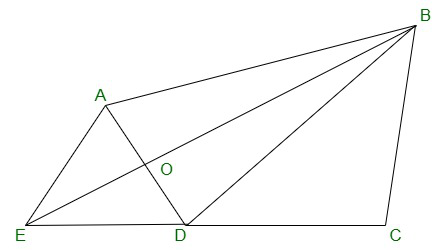We will get,

ΔBCE is the shape of Itwari’s original field

ΔAOB is the area for construction of the Health Centre.

ΔDEO is the land joined to the plot.

To prove:

ar(ΔDEO) = ar(ΔAOB)

Proof:

ΔDEB and ΔDAB lie on the same base BD, in-between the same parallels BD and AE.

Therefore,

Ar(ΔDEB) = ar(ΔDAB)

=> ar(ΔDEB) – ar(ΔDOB) = ar(ΔDAB) – ar(ΔDOB)

=> ar(ΔDEO) = ar(ΔAOB)

### [Hint: Join CX.]

Solution: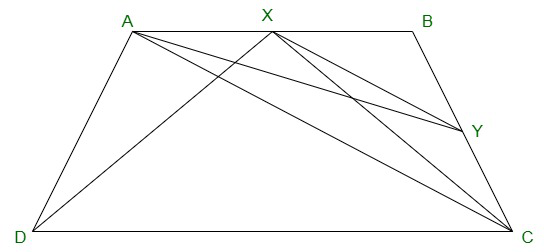Given:

ABCD is a trapezium with,

AB || DC.

XY || AC

To Construct,

Join CX

Prove:

Proof:

ar(ΔADX) = ar(ΔAXC)         -(equation 1)(Since they are on the same base AX and

in-between the same parallels AB and CD)

also,

ar(ΔAXC)=ar(ΔACY)          -(equation-2)(Since they are on the same base AC and

in-between the same parallels XY and AC)

From equation (1) and (2),

### Question 14. In Figure AP || BQ || CR. Prove that ar(ΔAQC) = ar(ΔPBR).

Solution: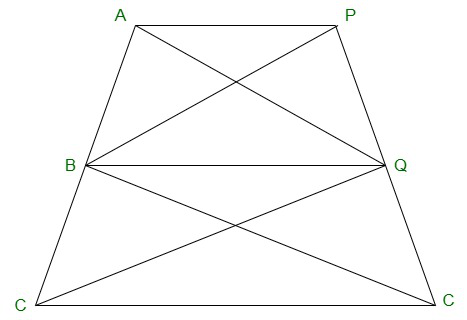Given:

AP || BQ || CR

Prove:

ar(AQC) = ar(PBR)

Proof:

ar(ΔAQB) = ar(ΔPBQ)          -(equation 1)(Since they are on the same base BQ and

between the same parallels AP and BQ)

also,

ar(ΔBQC) = ar(ΔBQR)          (equation 2)(Since they are on the same base BQ and

between the same parallels BQ and CR)

ar(ΔAQB)+ar(ΔBQC) = ar(ΔPBQ)+ar(ΔBQR)

=> ar(ΔAQC) = ar(ΔPBR)

### Question 15. Diagonals AC and BD of a quadrilateral ABCD intersect at O in such a way that ar(△AOD) = ar(△BOC). Prove that ABCD is a trapezium.

Solution: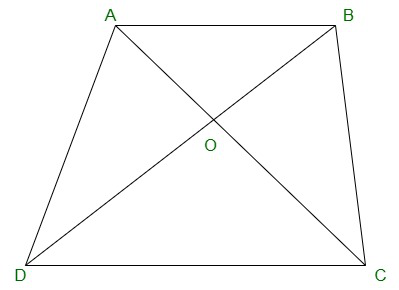Given:

ar(ΔAOD) = ar(ΔBOC)

Prove:

ABCD is a trapezium.

Proof:

ar(ΔAOD) = ar(ΔBOC)

=> ar(ΔAOD) + ar(ΔAOB) = ar(ΔBOC)+ar(ΔAOB)

Areas of ΔADB and ΔACB are equal.

Therefore,

They should be lying between the same parallel lines.

Therefore,

AB ∥  CD

Hence, ABCD is a trapezium.

### Question 16. In Figure, ar(DRC) = ar(DPC) and ar(BDP) = ar(ARC). Show that both the quadrilaterals ABCD and DCPR are trapeziums.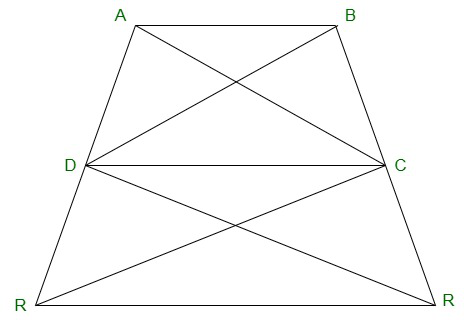Solution:

Given:

ar(ΔDRC) = ar(ΔDPC)

ar(ΔBDP) = ar(ΔARC)

Prove:

ABCD and DCPR are trapeziums.

Proof:

ar(ΔBDP) = ar(ΔARC)

⇒ ar(ΔBDP) – ar(ΔDPC) = ar(ΔDRC)

Therefore,

ar(ΔBDC) and ar(ΔADC) are lying in-between the same parallel lines.

Hence, AB ∥ CD

ABCD is a trapezium.

Similarly,

ar(ΔDRC) = ar(ΔDPC).

Therefore,

ar(ΔDRC) and ar(ΔDPC) are lying in-between the same parallel lines.

Hence, DC ∥ PR

Thus, DCPR is a trapezium.

My Personal Notes arrow_drop_up
Related Tutorials